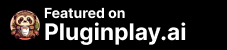# Wolfram Alpha

Sale price\$0.00## Wolfram Alpha AI app

### Access computation, math, curated knowledge & real-time data.

Why Install Wolfram Alpha AI to replace a human task?
Artificial Intelligence and Creativity Data and Analytics Education and Learning Language and Education Task and Project Management Utilities and Tools

## AI Information

### What is Wolfram Alpha

The Wolfram Alpha plugin for ChatGPT allows you to access the power of Wolfram Alpha directly from ChatGPT. Wolfram Alpha is a computational knowledge engine that can answer questions in a variety of topics, including math, science, history, and more.

To use the Wolfram Alpha plugin, you will need to first install it. You can do this by going to the ChatGPT website and clicking on the "Install Wolfram Alpha Plugin" button. Once you have installed the plugin, you will be able to access it by typing "/wolfram" in any ChatGPT chat.

Once you have accessed the Wolfram Alpha plugin, you can start using it to ask questions. For example, you could type "/wolfram what is the capital of France" and ChatGPT would respond with "The capital of France is Paris." You could also type "/wolfram what is the square root of 16" and ChatGPT would respond with "The square root of 16 is 4."

The Wolfram Alpha plugin is a powerful tool that can be used to improve your productivity and collaboration in ChatGPT. It is easy to use and can help you save time and effort.

Here are some of the features of the Wolfram Alpha plugin for ChatGPT:

Get answers: The Wolfram Alpha plugin can get answers to questions from Wolfram Alpha.
Perform calculations: The Wolfram Alpha plugin can perform calculations using Wolfram Language.
Easy to use: The Wolfram Alpha plugin is easy to use. Simply type in a question or request and ChatGPT will respond.
The Wolfram Alpha plugin is a powerful tool that can be used to improve your productivity and collaboration in ChatGPT. It is easy to use and can help you save time and effort.

Here are some examples of how you can use the Wolfram Alpha plugin for ChatGPT:

You can use the plugin to answer questions about a variety of topics. For example, you could type in "/wolfram what is the capital of France" and ChatGPT would respond with "The capital of France is Paris."
You can use the plugin to get answers to questions from Wolfram Alpha. For example, you could type in "/wolfram what is the square root of 16" and ChatGPT would respond with "The square root of 16 is 4."
You can use the plugin to perform calculations using Wolfram Language. For example, you could type in "/wolfram what is the area of a circle with a radius of 5" and ChatGPT would respond with "The area of a circle with a radius of 5 is 78.53981633974483."
The Wolfram Alpha plugin is a powerful tool that can be used to improve your productivity and collaboration in ChatGPT. It is easy to use and can help you save time and effort.

## TLDR: AI for Access computation, math, curated knowledge & real-time data. Copy and paste these prompts into Wolfram Alpha.

### Wolfram Alpha Prompts

#### Pluginplay prompts for Wolfram Alpha### Wolfram Alpha can be installed on

Wolfram Alpha - Official ChatGPT Plugin

### Who is Wolfram Alpha for?

The Wolfram Alpha plugin for ChatGPT can be used by a variety of users. Here are 5 potential users:

1. Students: Students can use the plugin to get answers to math and science problems, perform calculations, and research historical events, among other things.

2. Teachers: Teachers can use the plugin to create lesson plans, find resources for their classes, and answer questions from their students.

3. Researchers: Researchers can use the plugin to find data and information for their studies, perform calculations and analyses, and explore new ideas in their field.

4. Professionals: Professionals in fields such as finance, engineering, and medicine can use the plugin to perform calculations, research information, and stay up-to-date on developments in their industry.

5. General users: Anyone can use the plugin to get answers to questions, perform calculations, and explore new ideas and concepts.

Install Wolfram Alpha on ChatGPT Plugin Store

### What are the use cases for Wolfram Alpha?

1. Answering questions about various topics, such as math, science, history, and more.
2. Getting quick answers to questions from Wolfram Alpha.
3. Performing calculations using Wolfram Language.
4. Finding information about specific people, places, or events.
5. Converting units of measurement or currency.
6. Generating graphs or charts based on data.
7. Solving complex equations or problems.
8. Providing definitions for words or phrases.
9. Finding solutions to mathematical or scientific problems.
10. Generating random facts or trivia.